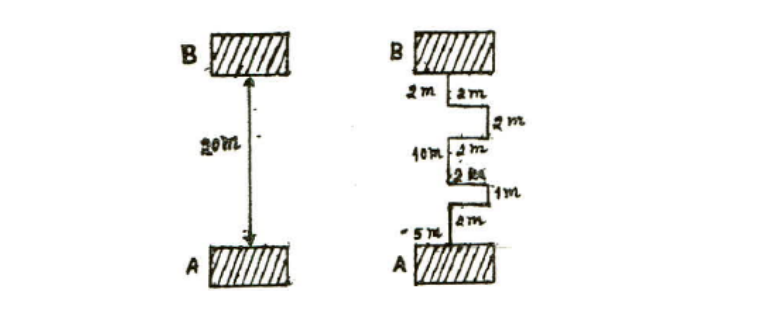# Why don't we just go straight?A $1\text{ kg}$ object is lifted from point A to point B through two different pathways as shown in the figure above. What are the respective amounts of work done in the left and right figures?

The gravitational acceleration is $g=9.8\text{ m/s}^2.$

×

Problem Loading...

Note Loading...

Set Loading...